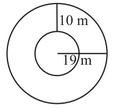# Q. 15.     Find the circumference of the inner and the outer circles, shown in the adjoining figure? (Take )G Gautam harsolia

We know that

Circumference of circle =

Now, the circumference of the inner circle  with radius (r) =  is

And the circumference of the outer circle  with radius (r ') = 19 m is

Therefore, the circumference of inner and outer circles are   respectively

Exams
Articles
Questions